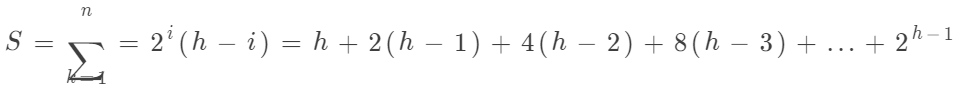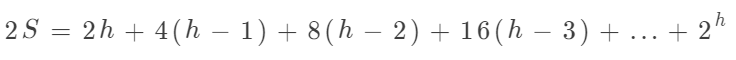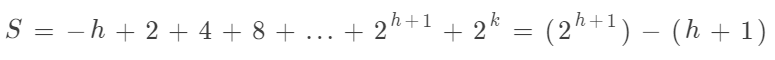# 面试题：求第K大元素（topK）[增强版]

### 一、引言

​ 这就是类似求Top（K）问题，什么意思呢？怎么在无序数组中找到第几（K）大元素？我们这里不考虑海量数据，能装入内存。

### 二、普通算法

#### 算法B:

1. 第一步：初始化长度为K的一个数组，先读入K个元素，将元素降序排序（升序也可以），这时候第K大元素就在最后一个。
2. 第二步：读入下一个元素就与已排序的第K大元素比较，如果大于，则将当前的第K大元素删掉，并将此元素放到前K-1个正确的位置上（这里就是简单的插入排序了。不了解插入排序的朋友可以看这里图解选择排序与插入排序）。
3. 时间复杂度：第一步采用普通排序算法时间复杂度是O(k^2)；第二步：(N-k)*(k-1) = Nk-k^2+k。所以算法B的时间复杂度为O(NK)当k=N/2（向下取整）时，时间复杂度还是O(n^2)。

### 三、较好算法

#### 算法C：

1. 算法思想：将数据读入一个数组，对数组进行buildHeap(我们这里构建大顶堆)，之后对堆进行K次deleteMax操作，第K次的结果就是我们需要的值。（因为是大顶堆，所以数据从大到小排了序，堆排序以后会详细说）。

2. 现在我们来解决上节遗留的问题，为什么buildHeap是线性的？不熟悉堆的可以看一下 图解优先队列（堆）。我们先来看看代码实现。

public PriorityQueue(T[] items) {       //当前堆中的元素个数       currentSize = items.length;       //可自行实现声明       array = (T[]) new Comparable[currentSize +1];       int i = 1;       for (T item : items){           array[i++] = item;       }       buildHeap();   }private void buildHeap() {       for (int i = currentSize / 2; i > 0; i--){           //堆的下滤方法，可参考上面的链接           percolateDown(i);       }   }

1. 什么叫满二叉树？满二叉树是完全填满的二叉树，最后一层都是填满的，如图中所示。完全二叉树，是除最后一层以外都是填满的，最后一层外也必须从左到右依次填入，就是上一篇中说的堆的结构。满二叉树一定是完全二叉树，完全二叉树不一定是满二叉树。

2. 证明定理：

容易看出，满二叉树中，高度为h上，有1个节点；高度h-1上2个节点，高度h-2上有2^2个节点及一般在高度h-i上的2i个节点组成。#### 算法D：

1. 算法思想：我们采用算法B的思想，只是我们这里构建一个节点数为K的小顶堆，只要下一个数比根节点大，就删除根节点，并将这个数进行下滤操作。所以算法最终的第K大数就是根节点的数。
2. 时间复杂度：对K个数进行buildHeap是O(k)，最坏情况下假设剩下的N-k个数都要进入堆中进行下滤操作，总的需要O(k+(N-k)logk)。如果K为N/2，则需要O(NlogN)。

#### 算法E：快速选择

• 算法思想：
1. 如果S中元素个数是1，那么k=1并将S中的元素返回。如果正在使用小数组的截止方法且|S|<=CUTOFF，则将S排序并返回第K个最大元素
2. 选取一个S中的元素v，称之为枢纽元（pivot）；
3. 将S-{v}（S中除了枢纽元中的其余元素）划分为两个不相交的集合S1和S2，S1集合中的所有元素小于等于枢纽元v，S2中的所有元素大于等于枢纽元；
4. 如果k<=|S1|，那么第k个最大元必然在S1中。这时，我们返回quickSelect(S1，k)。如果k=1+|S1|，那么枢纽元就是第k个最大元，我们直接返回枢纽元。否则，这第k个最大元一定在S2中，它就是S2中的第（k-|S1|-1）个最大元。我们进行一次递归调用并返回quickSelect(S2，k-|S1|-1)。
• java实现：
public class QuickSelect {    /**     * 截止范围     */    private static final int CUTOFF = 5;    public static void main(String[] args) {        Integer[] a = {8, 1, 4, 9, 6, 3, 5, 2, 7, 0, 12, 11, 15, 14, 13, 20, 18, 19, 17, 16};        int k = 5;        quickSelect(a, a.length - k + 1);        System.out.println("第" + k + "大元素是：" + a[a.length - k]);    }    public static <T extends Comparable<? super T>> void quickSelect(T[] a, int k) {        quickSelect(a, 0, a.length - 1, k);    }    private static <T extends Comparable<? super T>> void quickSelect(T[] a, int left, int right, int k) {        if (left + CUTOFF <= right) {            //三数中值分割法获取枢纽元            T pivot = median3(a, left, right);            //开始分割序列            int i = left, j = right - 1;            for (; ; ) {                while (a[++i].compareTo(pivot) < 0) {                }                while (a[--j].compareTo(pivot) > 0) {                }                if (i < j) {                    swapReferences(a, i, j);                } else {                    break;                }            }            //将枢纽元与位置i的元素交换位置            swapReferences(a, i, right - 1);            if (k <= i) {                quickSelect(a, left, i - 1, k);            } else if (k > i + 1) {                quickSelect(a, i + 1, right, k);            }        } else {            insertionSort(a, left, right);        }    }    private static <T extends Comparable<? super T>> T median3(T[] a, int left, int right) {        int center = (left + right) / 2;        if (a[center].compareTo(a[left]) < 0) {            swapReferences(a, left, center);        }        if (a[right].compareTo(a[left]) < 0) {            swapReferences(a, left, right);        }        if (a[right].compareTo(a[center]) < 0) {            swapReferences(a, center, right);        }        // 将枢纽元放置到right-1位置        swapReferences(a, center, right - 1);        return a[right - 1];    }    public static <T> void swapReferences(T[] a, int index1, int index2) {        T tmp = a[index1];        a[index1] = a[index2];        a[index2] = tmp;    }    private static <T extends Comparable<? super T>> void insertionSort(T[] a, int left, int right) {        for (int p = left + 1; p <= right; p++) {            T tmp = a[p];            int j;            for (j = p; j > left && tmp.compareTo(a[j - 1]) < 0; j--) {                a[j] = a[j - 1];            }            a[j] = tmp;        }    }}//输出结果//第5大元素是：16

• 最坏时间复杂度：与快速排序一样，当一个子序列为空时，最坏为O(N2)。
• 平均时间复杂度：可以看出，快速选择每次只用递归一个子序列。平均时间复杂度为O(N)。

### 四、总结

posted @ 2019-04-26 22:28  9龙  阅读(...)  评论(...编辑  收藏